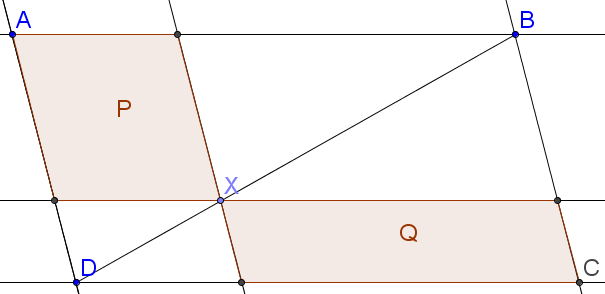# Interesting ParallelogramA point $X$ is drawn on the diagonal of a parallelogram $ABCD$. The lines parallel to the sides through $X$ are constructed, and quadrilaterals $P$ and $Q$ are formed, as shown in the diagram.

Given that $Q$ has base $12$ and perpendicular height $4$ and that the area of $ABCD$ is $200$, find the sum of all possible values of the base of $P$.

×